# Erlang distribution

(diff) ← Older revision | Latest revision (diff) | Newer revision → (diff)

The probability distribution concentrated on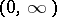with densitywhere the integer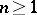and the real number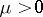are parameters. The characteristic function of the Erlang distribution has the formand the mathematical expectation and variance are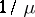and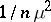, respectively.

The Erlang distribution is special case of the gamma-distribution:, where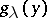is the density of the gamma-distribution for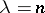, and where. Forthe Erlang distribution is the same as the exponential distribution with parameter. The Erlang distribution with parametersandcan be found as the distribution of the sum ofindependent random variables having the same exponential distribution with parameter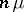. As, the Erlang distribution tends to the degenerate distribution concentrated at the point.

The selection of the Erlang distribution from the system of gamma-distributions is explained by its use in queueing theory. In many random queueing processes the Erlang distribution appears as the distribution of intervals among random events or as the distribution of the queueing time. Sometimes the Erlang distribution is defined as the gamma-distribution with the densityIt is named for A. Erlang, who was the first to construct mathematical models in queueing problems.

How to Cite This Entry:
Erlang distribution. Encyclopedia of Mathematics. URL: http://encyclopediaofmath.org/index.php?title=Erlang_distribution&oldid=14383
This article was adapted from an original article by A.V. Prokhorov (originator), which appeared in Encyclopedia of Mathematics - ISBN 1402006098. See original article